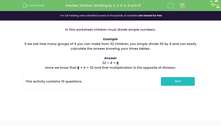# Division: Dividing by 2, 3, 4, 5, 8 and 10

In this worksheet, students divide simple numbers.Key stage:  KS 2

Curriculum topic:   Number: Multiplication and Division

Curriculum subtopic:   Use Multiplication/Division Facts (3, 4 and 8)

Popular topics:   Division worksheets

Difficulty level:#### Worksheet Overview

In this worksheet children must divide simple numbers.

Example

If we ask how many groups of 4 you can make from 32 children, you simply divide 32 by 4 and can easily calculate the answer knowing your times tables.

32 ÷ 4 = 8

since we know that 8 × 4 = 32 and that multiplication is the opposite of division.

### What is EdPlace?

We're your National Curriculum aligned online education content provider helping each child succeed in English, maths and science from year 1 to GCSE. With an EdPlace account you’ll be able to track and measure progress, helping each child achieve their best. We build confidence and attainment by personalising each child’s learning at a level that suits them.

Get started